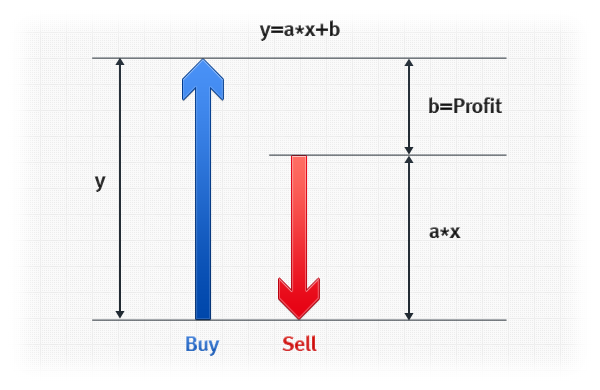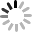购物车 (0)EA分类
• 黑盒B-Box
统计套利策略
统计套利策略
• 极影机器人
网格策略，盈利稳定
网格策略，盈利稳定
• 原油碰撞机
原油策略，经典对冲
原油策略，经典对冲
• 头皮风暴
头皮策略，闪电交易
头皮策略，闪电交易
• 百元EA
西点延迟EA（带Lmax账号）海龟趋势加仓EA评测
西点延迟EA（带Lmax账号）
海龟趋势加仓
EA评测
• 免费工具
跟单EA28货币EA切换MT4背景
跟单EA
28货币EA
切换MT4背景
• EA定制和VPS租用
EA定制，修改，VPS租用VPS租用服务
EA定制，修改，VPS租用
VPS租用服务

# 统计套利交易策略

### 经济调控手段

• 银行储备，例如累积的保险基金

• 进出口配额

• 补助在世界竞争中无法独立存活的某些经济领域

• 调节利率

### 利率

• 官方贴现率 (ODR) 是最有效的政府经济调节工具。这是央行对向其借钱的商业银行收取的利率。

• 回购利率是央行从商业银行统计和回购政府债券时使用的利率。

• 资金利率是储备金利率。

• 伦巴德利率是为抵押担保贷款收取的利率。

### 调控经济

• 投资于贩毒。结果是部分人口丧失劳动能力，犯罪率上升。

• 投资于泡沫。结果，金融停止作为经济生产和物品消耗的一部分，而变成彩票骗局的一部分。此类投资最终导致相当可观的一部分人失去储蓄。

• 投资于衍生品。衍生品是市场供需的去稳因素，带来显著的经济变化，最终导致世界危机。

### 统计套利交易的数学算法

1. 货币对的价格将向正掉期率方向变动。

2. 如果两个或更多货币对以高流通货币报价，则它们是正相关的。因此，可以通过反向的正相关仓位抵消价格变动。

v1 * d1 + v2 * d2 + …   + vn * dn   = profit

n 是金融工具的总数。
v1、v2、 …、vn 是要在相应金融工具中建立的持仓量。如果持仓量的值是负的，则建立卖出持仓。
d1、d2、 …、dn 是某个金融工具一个交易日内的平均价格变化。
profit 是一个交易日的平均盈利。

v1 * d1 + v2 * d2 = profit

d1   =   (-v2 * d2 + profit) / v1

v1 = 1
y = d1
a = -v2
b = profit

y = a * x + b

b’ = b – swap1 + a * swap2

swap1、swap2 是相应建仓方向上一个交易日内计算出来的货币对的掉期率。

1. 货币对的量和方向的选择使它们平均而言是盈利的。

2. 策略涉及的所有货币对的掉期率是正的。

### 范例y = 2 * x + 1

y – 2 * x = 1

### 实施

EA 计算两个金融工具中的持仓方向和量，从而获得平均盈利。然后，它请求服务器提供所选建仓方向的掉期率值的相关信息，并且在两个掉期率都是正的情况下，提供一个建议。

EA 源代码：

```//+------------------------------------------------------------------+//|                                        StatisticCarryTrading.mq5 |//|                                  Copyright 2012, Ruslan V. Lunev |//|                              https://www.mql5.com/ru/articles/491 |//+------------------------------------------------------------------+#property copyright "Copyright 2012, Ruslan V. Lunev"#property link      "https://www.mql5.com/ru/articles/491"#property version   "1.00"// Second currency pairinputstring secondpair="AUDUSD";
// Statistics collection period in barsinputint p=100;

// Arrays for storing historical opening pricesdouble open0[];
double open1[];

//+------------------------------------------------------------------+//| Expert initialization function                                   |//+------------------------------------------------------------------+intOnInit()
{
// Reading the time series of opening prices
// for the currency pairs involved
CopyOpen(_Symbol,PERIOD_D1,0,p+1,open0);
ArraySetAsSeries(open0,true);
CopyOpen(secondpair,PERIOD_D1,0,p+1,open1);
ArraySetAsSeries(open1,true);

int i=0;

double pp=p;

double s1 = 0;
double s2 = 0;
double s3 = 0;
double s4=open1-open1[p];
double s5=open0-open0[p];

double averagex = s4 / pp;
double averagey = s5 / pp;

for(i=0; i<p; i++)
{
double x0 = open1[i] - open1[i + 1];
double y0 = open0[i] - open0[i + 1];
double x1 = x0 - averagex;
double y1 = y0 - averagey;
s1 = s1 + x1 * x1;
s2 = s2 + y1 * y1;
s3 = s3 + x1 * y1;
}

// Pearson's linear correlation coefficient
double r=s3/MathSqrt(s1*s2);

// Calculation of proportions of opening positions sizes given the contract sizes
double a = signum(r) * SymbolInfoDouble(_Symbol, SYMBOL_TRADE_CONTRACT_SIZE) * MathSqrt(s2)

// Calculation of the average daily profit
double b = averagey - averagex * a;

// Derive the resulting formula of joint price movement
if(b>0)
{
Print(_Symbol+" = ",a," * "+secondpair+" + ",b);
} else {
Print(_Symbol+" = ",a," * "+secondpair+" - ",MathAbs(b));
}

a=-a*signum(b);

// Recommendations

if(b<0)
{
recomendation="Sell "+_Symbol;
if(SymbolInfoDouble(_Symbol,SYMBOL_SWAP_SHORT)<0.0)
{
recomendation="Short positions swap in "+_Symbol+" is negative";
MessageBox(recomendation,"Not recommended",1);
return(0);
}
} else {
if(SymbolInfoDouble(_Symbol,SYMBOL_SWAP_LONG)<0.0)
{
recomendation="Long positions swap in "+_Symbol+" is negative";
MessageBox(recomendation,"Not recommended",1);
return(0);
}
}

if(a<0)
{
recomendation=recomendation+"\r\nSell "+a+" "+secondpair;
if(SymbolInfoDouble(secondpair,SYMBOL_SWAP_SHORT)<0.0)
{
recomendation="Short positions swap in "+secondpair+" is negative";
MessageBox(recomendation,"Not recommended",1);
return(0);
}
} else {
if(SymbolInfoDouble(secondpair,SYMBOL_SWAP_LONG)<0.0)
{
recomendation="Long positions swap in "+secondpair+" is negative";
MessageBox(recomendation,"Not recommended",1);
return(0);
}
}

double profit=MathAbs(b)/SymbolInfoDouble(_Symbol,SYMBOL_POINT);

if((SymbolInfoInteger(_Symbol,SYMBOL_DIGITS)==5) || (SymbolInfoInteger(_Symbol,SYMBOL_DIGITS)==3))
{
profit=profit/10;
}

recomendation = recomendation + "\r\nCorrelation coefficient: " + r;
recomendation = recomendation + "\r\nAverage daily profit: "
+ profit + " points";

MessageBox(recomendation,"Recommendation",1);

return(0);
}

// Step function - Signumdouble signum(double x)
{
if(x<0.0)
{
return(-1.0);
}
if(x==0.0)
{
return(0);
}
return(1.0);
}
//+-----------------------The End ------------------------```

EA 有两个输入参数：

• p 是日柱的周期，它提供计算所需的所有统计。此参数的值最好不要超过他们的三种货币列在第一个和第二个货币对中的国家的央行最后一次改变贴现率的时间。

• secondpair 是第二个金融工具。第一个金融工具是 EA 附加到的图表的货币对。选择第二个货币对是让用于计算点数的货币与第一个货币对中用于计算点数的货币一致（货币对标识符中的最后三个字符）。例如：EURUSD 和 AUDUSD 或 GBPJPY 和 NZDJPY 等。在选择第二个货币对之前，应通过检查交易一览表来确保它有正的掉期率。

EA 应附加到第一个货币对运行在 D1 时间框架的图表上，而第二个货币对应在输入参数中指定。

### 警告7X15小时客户服务

EA配送

QQ或者微信发送
2小时内送达苏公网安备 32011302320766号

0